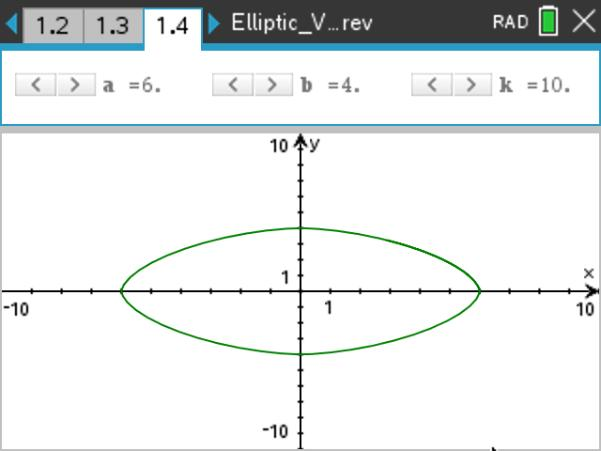••• ##### Device
•TI-Nspire™ CX/CX II
•TI-Nspire™ CX CAS/CX II CAS
• TI-Nspire™ Navigator™
• ##### Software

TI-Nspire™ CX
TI-Nspire™ CX CAS

5.0

• ##### Report an Issue

Precalculus: Elliptic Variations
by Texas Instruments#### Objectives

• Students will be able to describe the characteristics of the graphs of equations of the form |(x/a)|n + |(y/b)|n = 1 for various values of n.
• Students will be able to describe the characteristics of the graphs of equations of the form √((x-a)2+y2)*√((x+a)2+y2) = b2 when a = 4 and b varies.

• ellipse
• superellipse
• Cassini oval

#### About the Lesson

This lesson involves exploring the curves that result from varying the defining conditions of an ellipse.
As a result, students will:

• Analyze the properties of the graphs and equations of superellipses and Cassini ovals.
• Analyze the derivations of the parametric representation of a superellipse and the polar representation of a Cassini oval.